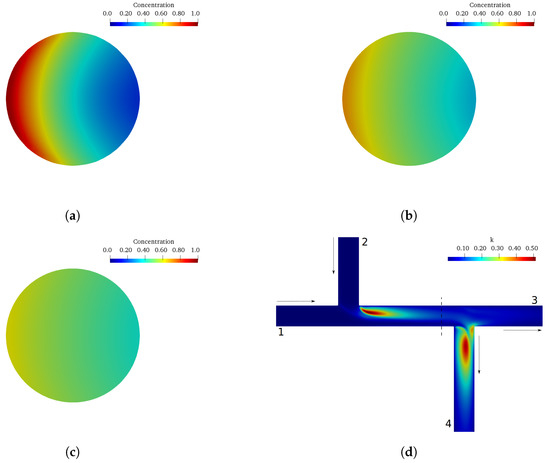Grbčić, L., Kranjčević, L., Lučin, I., Čarija, Z.

Efficient Double-Tee Junction Mixing Assessment by Machine Learning
Water, (2020), doi.org/10.3390/w12010238, (quartile Q1)

Abstract: A new approach in modeling of mixing phenomena in double-Tee pipe junctions based on machine learning is presented in this paper. Machine learning represents a paradigm shift that can be efficiently used to calculate needed mixing parameters. Usually, these parameters are obtained either by experiment or by computational fluid dynamics (CFD) numerical modeling. A machine learning approach is used together with a CFD model. The CFD model was calibrated with experimental data from a previous study and it served as a generator of input data for the machine learning metamodels—Artificial Neural Network (ANN) and Support Vector Regression (SVR). Metamodel input variables are defined as inlet pipe flow ratio, outlet pipe flow ratio, and the distance between the pipe junctions, with the output parameter being the branch pipe outlet to main inlet pipe mixing ratio. A comparison of ANN and SVR models showed that ANN outperforms SVR in accuracy for a given problem. Consequently, ANN proved to be a viable way to model mixing phenomena in double-Tee junctions also because its mixing prediction time is extremely efficient (compared to CFD time). Because of its high computational efficiency, the machine learning metamodel can be directly incorporated into pipe network numerical models in future studies.